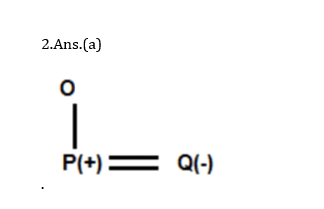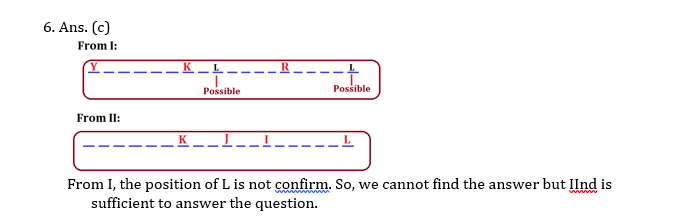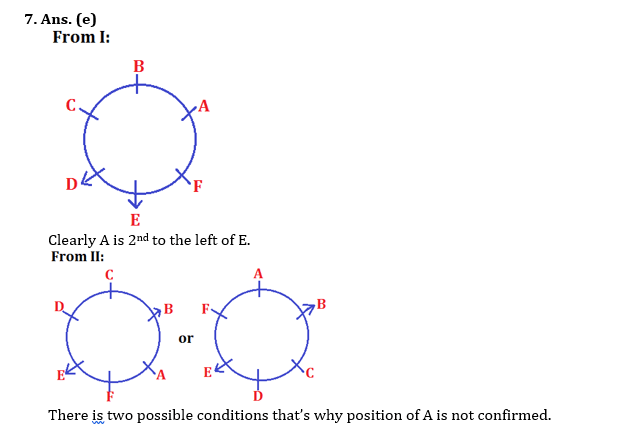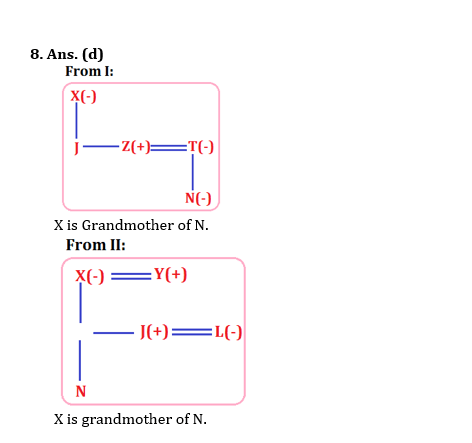# Data Sufficiency for IBPS, SBI, RBI, RRBs: Quiz 5

## Data Sufficiency for IBPS, SBI, RBI, RRBs: Quiz 5

Data Sufficiency plays a significant role in Reasoning Ability Section of banking exams such as IBPS, SBI and RBI PO and Clerk. You will get at least 5 questions from Data Sufficiency in one of IBPS, SBI and RBI PO & clerk prelims and Mains exam. So, aspirants should focus on Data Sufficiency questions in detail. Here, we are providing you with the Data Sufficiency questions quiz with the detailed solution so that you can easily prepare for Data Sufficiency questions. We are providing here all-important latest pattern-based questions and Previous Year Questions of Data Sufficiency of various Government Exam like IBPS, SBI, and RBI PO and Clerk exam. This Data Sufficiency quiz we are providing is free. Attempt this Data Sufficiency quiz to practice important questions with answers and solutions. And score better in IBPS, SBI and RBI PO and Clerk exam.

Data Sufficiency Quiz to improve your Reasoning Ability for SBI Po & SBI clerk exam IBPS PO Reasoning, IBPS Clerk Reasoning, IBPS RRB Reasoning, LIC AAO, LIC Assistant and another competitive exam.

Directions (1-5): Each of the questions below consists of a question and three statements numbered I, and II given below it. You have to decide whether the data provided in the statements are sufficient to answer the question. Read all the statements and answer the following questions.

(a) If the data in statement I alone is sufficient to answer the question.

(b) If the data in statement II alone is sufficient to answer the question.

(c) If the data either in statement I alone or statement II alone are sufficient to answer the question.

(d)If the data given in both I and II together are not sufficient to answer the question.

(e) If the data in both the statements I and II together are necessary to answer the question.

Q1. What does ‘na’ means in a code language?

I. ‘pa naja’ means ‘The Amanmahesh’ in the code language.

II. ‘hoka pa’ means ‘ankitamaheshankit’ in the code language.

1.Ans.(d)

Q2. How is Q related to O ?

I. P is Son of O and husband of Q.

II. O is mother of R who is brother of P.Q3. How many students among P, Q, R and S have passed the examination?

I. The following is a true statement: P and Q passed the examination.

II. The following is a false statement: One among R and S has passed the examination.

3.Ans.(d)

From statement I, P & Q have passed. But nothing about R & S. From statements II , ‘One among R and S has passed’ and this is false. This indicates ‘R and S both have passed or both are failed. Hence we get both only P and Q have passed or all P, Q, R, S have passed.

Q4. The last Sunday of March, 2013 fell on which date?

I. The first Sunday of that month fell on 5th.

II. The last day of that month was Friday.

4.Ans.(c)

Using each statement alone, we can find that the last Sunday of March 2013 was on 26th March.

Q5.  Among six persons K, J, I, H, G and F who are standing around a circle, some of them are facing the centre while others are facing outside (i.e. opposite to the centre,). What is the position of K with respect to G?

(Note: Facing the same direction means, if one is facing the centre then the other is also facing the centre and vice versa. Facing the opposite directions means, if one is facing the centre then the other is facing outside and vice versa).

I. I stands second to the right of G. G faces outside. I is an immediate neighbour of both H and J. F stands second to the left of H . H  faces the same direction as G.

II. Only two persons stand between J and G. Both J and G face outside. G is an immediate neighbour of both H and F. J is an immediate neighbour of both I and K. K is not an immediate neighbour of H.Directions (6-8): These questions consist of a question and two statements number I and II given below, you have to decide whether the data given in the statements are sufficient to answer the question. Read both the statements and choose the most appropriate option.

Q6. How many people are standing between L and K in a straight line of 19 people?

(Note- All are standing in a straight line facing north)

I. Y stands to the extreme left end of the line. Only five people stand between Y and K. Only six people stand between K and R. Only four people stand between R and L.

II. J stands exactly in the centre of the line. Only two people stand between I and J. Only five people stand between I and L. I stand to the left of L. K stands third to the left of J.

(a) The data even in both statements I and II together are not sufficient to answer the question.

(b) The data in both statements I and II together are necessary to answer the question.

(c) The data in statement II alone are sufficient to answer the question while the data in statement I alone are not sufficient to answer the question.

(d) The data either in statement I alone or statement II alone are sufficient to answer the question.

(e) The data in statement I alone are sufficient to answer the question while the data in statement II alone are not sufficient to answer the question.Q7. Among six people A, B, C, D, E and F standing around a circle. Some facing the centre while some facing outside (i.e., opposite to the centre) what is the position of A with respect to E?

(Note -Facing the same direction means, if one is facing the centre, then the other also faces the centre and vice-versa. Facing the opposite direction means, if one is facing the centre, then the other faces outside and vice-versa).

1. C stands second to the right of E. E faces outside. C is an immediate neighbor of both D and B. F stands second to the left of D. D faces the same direction as E.
2. Only two people stand between B and E. Both B and E face outside. E is an immediate neighbor of both D and F. B is an immediate neighbor of both C and A. A is not an immediate neighbor of D.

(a) The data in both statements I and II together are necessary to answer the question.

(b) The data in statement II alone are sufficient to answer the question while the data in statement I alone are not sufficient to answer the question.

(c) The data even in both statements I and II together are not sufficient to answer the question.

(d) The data either in statement I alone or statement II alone are sufficient to answer the question.

(e) The data in statement I alone are sufficient to answer the question while the data in statement II alone are not sufficient to answer the question.Q8. How is X related to N?

1. X is the mother of J. T is married to Z. N is the daughter of T. Z is the brother of J.
2. X is married to Y. Y is the father of J. J is married to L. J is the paternal uncle of N.

(a) The data even in both statements I and II together are not sufficient to answer the question.

(b) The data in statement I alone are sufficient to answer the question while the data in statement II alone are not sufficient to answer the question.

(c) The data either in statement I alone or statement II alone are sufficient to answer the question.

(d) The data in both statements I and II together are necessary to answer the question.

(e) The data in statement II alone are sufficient to answer the question while the data in statement I alone are not sufficient to answer the question.Directions (9–10): Each of the questions below consists of a question and two statements numbered I and II given below it. You have to decide whether the data provided in the statements are sufficient to answer the question. Read both the statements.

(a) If the data in statement I alone are sufficient to answer the question, while the data in statement II alone are not sufficient to answer the question

(b) If the data in statement II alone are sufficient to answer the question, while the data in statement I alone are not sufficient to answer the question

(c) If the data either in statement I alone or in statement II alone are sufficient to answer the question

(d) If the data in both statements I and II together are not sufficient to answer the question

(e) If the data in both statements I and II together are necessary to answer the question

Q9. How is Pawan related to Raman?

I. Pawan is the grandson of Raman’s father.

II. Raman has no sister but has several friends and each of his friends is the only son in his respective family.

1. Ans.(d)

From I: We get Pawan is either nephew or son of Raman so we can’t say what is the relation between Pawan and Raman.

From II: The information does not give the clue to reach answer.

So both I and II are not sufficient to answer the question.

Q10. In which direction is car A with respect to car D?

I. Car D is in south of car B, which is in west of car C.

II. Car A is in south-east of car B.

1. Ans.(d)

From both the statements we cannot find the direction of car A with respect to car D.

#### Aatma Nirbhar Reasoning Topic Wise Online Test Series

Recommended PDF’s for:

#### Most important PDF’s for Bank, SSC, Railway and Other Government Exam : Download PDF Now

AATMA-NIRBHAR Series- Static GK/Awareness Practice Ebook PDF Get PDF here
The Banking Awareness 500 MCQs E-book| Bilingual (Hindi + English) Get PDF here
AATMA-NIRBHAR Series- Banking Awareness Practice Ebook PDF Get PDF here
Computer Awareness Capsule 2.O Get PDF here
AATMA-NIRBHAR Series Quantitative Aptitude Topic-Wise PDF Get PDF here
AATMA-NIRBHAR Series Reasoning Topic-Wise PDF Get PDF Here
Memory Based Puzzle E-book | 2016-19 Exams Covered Get PDF here
Caselet Data Interpretation 200 Questions Get PDF here
Puzzle & Seating Arrangement E-Book for BANK PO MAINS (Vol-1) Get PDF here
ARITHMETIC DATA INTERPRETATION 2.O E-book Get PDF here
3